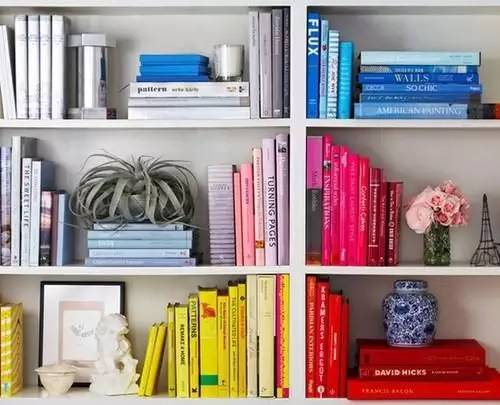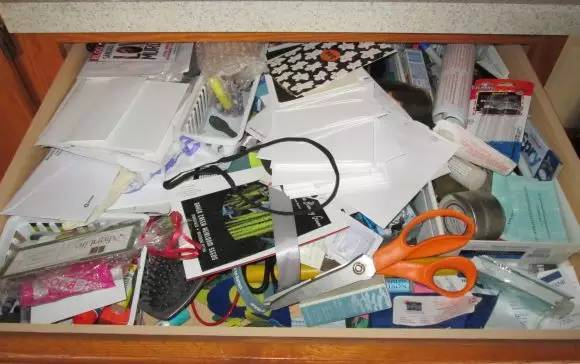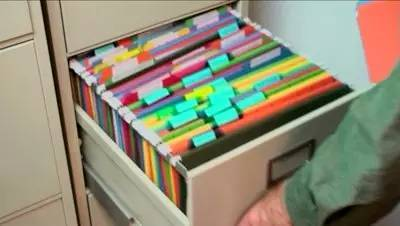|

# 买书如山倒,读书如抽丝?你的问题出在不做整理

我做过不少整理收纳的讲座、咨询（你也可以在知乎上找到我，知乎 ID：敬子敬子），很多人跟我说家里最难处理的是那一大柜子衣服，我反倒觉得书和文件才是最难收拾的。

因为它们体积小，散落在家中各处（随便翻翻你的包包、抽屉甚至是大衣口袋，是不是有那么几张不知何时装进去的收据？），但又不像杂物零碎一样让房间有直观的杂乱感，所以往往让人掉以轻心，以为会很好处理，结果操作起来，整理不彻底、收纳不高效。那么，究竟该怎么处理书和文件呢？真的要把不看、不用的书都丢掉，把所有文件一份一份整理归档？别急，咱们一样一样来说。

什么时候需要整理书？

书是非常特殊的物品。它不只是工具，有时还会被附加精神、情感因素。不少人有藏书的习惯，很担心做整理就一定要扔书，心理上有抵抗。

然而，书的整理原则并非“扔扔扔”。举个栗子：

有一位跟我约了上门整理咨询的小伙伴，在我到他家之前就很担心地问：“老师，我的书有很多本是不看的，放在家里就是用来装点门面的，你会不会劝我扔了？”我跟他确认说：“你非常清楚你是用来装点门面的吗？”他说“是”，我点头，那没问题，目的清晰、需求清晰，没必要扔啊！

这说明了一个基本原则，就是我们得清晰地知道自己收藏书的目的和需求。村上春树的书房，书架“顶天立地”，里头的书可能包过书皮，一眼看去几乎都是白色的。

书的整理有一个以人为本的原则。藏书爱书之人，往往有自己的取舍和摆放逻辑，按照这个逻辑来整理、收纳就好。有的人对书籍已经有了清晰的选购、评分、淘汰、排列的原则，并且有靠谱、可执行的阅读计划，那么通常我倾向于尊重并保留他现有的原则，如果他自己没有感觉到不舒适，就不用特意解决这个问题。

如果你对书籍购买、阅读、淘汰的原则不是很明确，而且出现了以下情况，那么就需要整理了：

书籍存量到达空间上限。摆不下，放不了，家里的书散落在沙发、书柜、书桌、厕所、床头柜等各处。好多书不看了、不用了，懒得处理，扔了似乎好可惜，不处理又觉得占地方。

屯书不读，造成极大的心理压力。买书如山倒，读书如抽丝。好些书计划要看，一直排不出时间；或者一旦想学新东西，就火速先买几本书，结果拖拖拉拉，迟迟没有安排出计划来看，产生心理压力。

模糊崇拜，无意识管理。根深蒂固地觉得什么都可以扔，但是书这样的东西是不能扔的，对书有精神崇拜和依赖，但并无清晰管理的方法。

书籍：该扔就扔，别自我欺骗

当你确认需要进行书籍整理时，就赶紧动手吧。

整理书的时候，我们需要把书全部集中，一本一本判断决策是否保留。在判断的时候，不要打开翻阅，否则整理的过程中一旦看起了兴致，很可能注意力就涣散了，导致最后翻来翻去，几个小时过去了，整理还迟迟结束不了。

整理书的原则，可参考以下几条：

属于他人，或者转送他人的书，应该拿出来立刻快递给对方，不要拖延。

有情感记忆的书应该拿出来，与其他回忆物品一起整理或保存。

书中有部分内容特别想保存的，立刻剪报、拍照或者整理到自己的笔记中。

不再看、不再用的书，应当扔掉。

一年没有看的书，建议扔掉。尤其要问问自己为什么没有看，探究这个真相很重要。

对于打算要看的书，当场制定出计划。计划可以以 1 个月或者 3 个月为期限来制定，如果到期无法执行，就放弃。屯书而无阅读计划，纯属自我欺骗。最后留下来的书，如果还有腰封的，丢掉腰封后将书集中收纳在一类家具里，比如书柜。分类和排列的原则，因个人喜好而定，按颜色、高矮、流派、学科去分类都行。

文件：基本原则是全扔

这里说的文件不包括电子文件，也不完全包括职场上的文件，主要是指在家庭生活中出现的各类小票、发票、收据、卡、合同、说明书、保修单、证件等。跟书相比，这些文件占的空间更小，但是种类更加纷繁复杂，难于整理。你家也一定有这么个抽屉，堆满了各种票据杂物。

由于文件是重要的信息载体，在安全感驱使下，大家都想尽量保留它们。因此，我们常常看到这样的现象：家用电器早已淘汰，保修卡、说明书竟然还留着；小票、收据打算留下来记账，但散乱堆叠，最终也没有记成账；各类会员卡、购物卡、积分卡等把钱包撑得鼓鼓的；合同、发票都不敢扔，但用的时候又找不到……

那该怎么办？

❶ 先分类整理，减轻心理压力

纸质文件的整理，我们应当抱着“尽量全扔”的想法。这想法乍一听让人非常没有安全感，对，就是逼着你走出心理舒适区。你可以通过分类处理的办法来减轻心理压力：

把当下手头正在用的，或者短时期内需要用的文件拿出来保存。

万一扔掉会非常困扰的资产、身份证明、资历证明文件，例如身份证、户口本、房产证、结婚证、驾照、合同、学位学历证等，这些已经进入贵重物品范畴，应当拎出来和其他贵重物品一起保存。

情感记忆文件。在学习、工作、生活过程中，还会产生一些想要保存的文件，例如奖状、聘书等，这部分如果不具有贵重物品性质，但有情感回忆、想要保存，那就应当与回忆物品一起保存。待报销的票据用夹子或者文件袋收纳好。

❷ 尽量全扔，扔不了的再做收纳

剩下的文件我们就抱着“尽量全扔”的态度，扔掉、碎掉或者电子化之后扔掉。有一些具体操作小贴士供大家参考：

有电子版的文件就不保存纸版。项目成果文件尽量保存终稿。

在整理的时候，如果你要保留文件，同时不喜欢外壳的话，外壳也可以扔。比如我的学位证书，外壳都扔掉了，只保留内文文件。

切记不要保留不在自己工作范围和职责范围之内的文件。不要帮领导和同事囤积物品。请仔细思考你手上的文件究竟是你的，还是别人的。如果你整理物品时，发现有别人的文件，这是个好机会，说明你的工作界限不清，应该感谢整理带给你的启发，解决一下这个问题。

文件没有了之后，文件的收纳用品也可以扔掉，比如名片盒。

算算看有多少文件，再买个合适且心动的文件夹收纳。不要多买文件夹。可以腾出一个抽屉专门收纳各类文件。

这里就不给大家推荐具体的收纳产品了，相信长尾夹、文件夹这样的东西，你家里多少有一些，而且整理收纳后，留下的文件也不会多，家里已有的收纳用品就足够用了，不必多买新的。

❸ 票据、会员卡、名片等这么处理

根据以上两点整理收纳方法，家里最常出现的发票单据、购物小票、各种卡片、名片等就可以放心大胆地这么处理了：

发票单据：需要一个时间段才能报销的发票，单独整理出来，装入发票盒子或拿长尾夹夹着。其他的全扔。

购物小票：全扔。如果需要记账，记账之后全部扔掉。如果没有记账习惯，就不要自己欺骗自己了。

会员卡、购物卡、积分卡：拍照或者保留在手帐、笔记类 App 里以后，全扔。商家使用时报卡号就可以了。如果你不放心，不要拖延，立刻给所有商家打电话确认是否一定要使用实体卡，绝大多数商家都可以报卡号使用。以后拿卡的时候也记得要问。

说明书、保修卡：说明书建议全扔，因为一般都能在其官网上找到电子版的说明书。保修卡统一收入文件袋后分类。

名片：要联系的人早联系了，不联系的人到了对方离职跳槽也不会有联系。要是产生了“万一有一天要用了怎么办”的焦虑，那就思考这个万一究竟指什么，是什么情景。对将来发生的不确定的事情，尽量具体化。实际上，如果未来有极大的需要，要去找到一个人的联系方式，怎么样都能通过社交圈找到对口的人。如果岗位需要对接很多人，每天、每周都会交换到新的名片，就要注意建立起一套工作圈人际信息处理办法，而不要无意识不管理，最后名片堆成山。有些朋友喜欢用漂亮的名片夹存放名片，似乎这个过程就很有成就感。然而，名片并不是工作的成果哦，它们代表的只是工作的过程。对书的整理，其实是明晰自己的阅读、学习规划；对文件的整理，其实是对自己生活的删繁就简。找个时间，好好整理一番吧！

转载已获作者授权，著作权归作者所有。

商业转载请联系作者获得授权，非商业转载请注明出处。

来源：清单（清单APP下载链接）

阅读原文`声明：本文由入驻焦点开放平台的作者撰写，除焦点官方账号外，观点仅代表作者本人，不代表焦点立场错误信息举报电话： 400-099-0099，邮箱：jubao@vip.sohu.com，或点此进行意见反馈，或点此进行举报投诉。`A B C D E F G H J K L M N P Q R S T W X Y Z
A - B - C - D - E
• A
• 鞍山
• 安庆
• 安阳
• 安顺
• 安康
• 澳门
• B
• 北京
• 保定
• 包头
• 巴彦淖尔
• 本溪
• 蚌埠
• 亳州
• 滨州
• 北海
• 百色
• 巴中
• 毕节
• 保山
• 宝鸡
• 白银
• 巴州
• C
• 承德
• 沧州
• 长治
• 赤峰
• 朝阳
• 长春
• 常州
• 滁州
• 池州
• 长沙
• 常德
• 郴州
• 潮州
• 崇左
• 重庆
• 成都
• 楚雄
• 昌都
• 慈溪
• 常熟
• D
• 大同
• 大连
• 丹东
• 大庆
• 东营
• 德州
• 东莞
• 德阳
• 达州
• 大理
• 德宏
• 定西
• 儋州
• 东平
• E
• 鄂尔多斯
• 鄂州
• 恩施
F - G - H - I - J
• F
• 抚顺
• 阜新
• 阜阳
• 福州
• 抚州
• 佛山
• 防城港
• G
• 赣州
• 广州
• 桂林
• 贵港
• 广元
• 广安
• 贵阳
• 固原
• H
• 邯郸
• 衡水
• 呼和浩特
• 呼伦贝尔
• 葫芦岛
• 哈尔滨
• 黑河
• 淮安
• 杭州
• 湖州
• 合肥
• 淮南
• 淮北
• 黄山
• 菏泽
• 鹤壁
• 黄石
• 黄冈
• 衡阳
• 怀化
• 惠州
• 河源
• 贺州
• 河池
• 海口
• 红河
• 汉中
• 海东
• 怀来
• I
• J
• 晋中
• 锦州
• 吉林
• 鸡西
• 佳木斯
• 嘉兴
• 金华
• 景德镇
• 九江
• 吉安
• 济南
• 济宁
• 焦作
• 荆门
• 荆州
• 江门
• 揭阳
• 金昌
• 酒泉
• 嘉峪关
K - L - M - N - P
• K
• 开封
• 昆明
• 昆山
• L
• 廊坊
• 临汾
• 辽阳
• 连云港
• 丽水
• 六安
• 龙岩
• 莱芜
• 临沂
• 聊城
• 洛阳
• 漯河
• 娄底
• 柳州
• 来宾
• 泸州
• 乐山
• 六盘水
• 丽江
• 临沧
• 拉萨
• 林芝
• 兰州
• 陇南
• M
• 牡丹江
• 马鞍山
• 茂名
• 梅州
• 绵阳
• 眉山
• N
• 南京
• 南通
• 宁波
• 南平
• 宁德
• 南昌
• 南阳
• 南宁
• 内江
• 南充
• P
• 盘锦
• 莆田
• 平顶山
• 濮阳
• 攀枝花
• 普洱
• 平凉
Q - R - S - T - W
• Q
• 秦皇岛
• 齐齐哈尔
• 衢州
• 泉州
• 青岛
• 清远
• 钦州
• 黔南
• 曲靖
• 庆阳
• R
• 日照
• 日喀则
• S
• 石家庄
• 沈阳
• 双鸭山
• 绥化
• 上海
• 苏州
• 宿迁
• 绍兴
• 宿州
• 三明
• 上饶
• 三门峡
• 商丘
• 十堰
• 随州
• 邵阳
• 韶关
• 深圳
• 汕头
• 汕尾
• 三亚
• 三沙
• 遂宁
• 山南
• 商洛
• 石嘴山
• T
• 天津
• 唐山
• 太原
• 通辽
• 铁岭
• 泰州
• 台州
• 铜陵
• 泰安
• 铜仁
• 铜川
• 天水
• 天门
• W
• 乌海
• 乌兰察布
• 无锡
• 温州
• 芜湖
• 潍坊
• 威海
• 武汉
• 梧州
• 渭南
• 武威
• 吴忠
• 乌鲁木齐
X - Y - Z
• X
• 邢台
• 徐州
• 宣城
• 厦门
• 新乡
• 许昌
• 信阳
• 襄阳
• 孝感
• 咸宁
• 湘潭
• 湘西
• 西双版纳
• 西安
• 咸阳
• 西宁
• 仙桃
• 西昌
• Y
• 运城
• 营口
• 盐城
• 扬州
• 鹰潭
• 宜春
• 烟台
• 宜昌
• 岳阳
• 益阳
• 永州
• 阳江
• 云浮
• 玉林
• 宜宾
• 雅安
• 玉溪
• 延安
• 榆林
• 银川
• Z
• 张家口
• 镇江
• 舟山
• 漳州
• 淄博
• 枣庄
• 郑州
• 周口
• 驻马店
• 株洲
• 张家界
• 珠海
• 湛江
• 肇庆
• 中山
• 自贡
• 资阳
• 遵义
• 昭通
• 张掖
• 中卫

1室1厅1厨1卫1阳台

1
2
3
4
5

0
1
2

1

1

0
1
2
3报名成功，资料已提交审核A B C D E F G H J K L M N P Q R S T W X Y Z
A - B - C - D - E
• A
• 鞍山
• 安庆
• 安阳
• 安顺
• 安康
• 澳门
• B
• 北京
• 保定
• 包头
• 巴彦淖尔
• 本溪
• 蚌埠
• 亳州
• 滨州
• 北海
• 百色
• 巴中
• 毕节
• 保山
• 宝鸡
• 白银
• 巴州
• C
• 承德
• 沧州
• 长治
• 赤峰
• 朝阳
• 长春
• 常州
• 滁州
• 池州
• 长沙
• 常德
• 郴州
• 潮州
• 崇左
• 重庆
• 成都
• 楚雄
• 昌都
• 慈溪
• 常熟
• D
• 大同
• 大连
• 丹东
• 大庆
• 东营
• 德州
• 东莞
• 德阳
• 达州
• 大理
• 德宏
• 定西
• 儋州
• 东平
• E
• 鄂尔多斯
• 鄂州
• 恩施
F - G - H - I - J
• F
• 抚顺
• 阜新
• 阜阳
• 福州
• 抚州
• 佛山
• 防城港
• G
• 赣州
• 广州
• 桂林
• 贵港
• 广元
• 广安
• 贵阳
• 固原
• H
• 邯郸
• 衡水
• 呼和浩特
• 呼伦贝尔
• 葫芦岛
• 哈尔滨
• 黑河
• 淮安
• 杭州
• 湖州
• 合肥
• 淮南
• 淮北
• 黄山
• 菏泽
• 鹤壁
• 黄石
• 黄冈
• 衡阳
• 怀化
• 惠州
• 河源
• 贺州
• 河池
• 海口
• 红河
• 汉中
• 海东
• 怀来
• I
• J
• 晋中
• 锦州
• 吉林
• 鸡西
• 佳木斯
• 嘉兴
• 金华
• 景德镇
• 九江
• 吉安
• 济南
• 济宁
• 焦作
• 荆门
• 荆州
• 江门
• 揭阳
• 金昌
• 酒泉
• 嘉峪关
K - L - M - N - P
• K
• 开封
• 昆明
• 昆山
• L
• 廊坊
• 临汾
• 辽阳
• 连云港
• 丽水
• 六安
• 龙岩
• 莱芜
• 临沂
• 聊城
• 洛阳
• 漯河
• 娄底
• 柳州
• 来宾
• 泸州
• 乐山
• 六盘水
• 丽江
• 临沧
• 拉萨
• 林芝
• 兰州
• 陇南
• M
• 牡丹江
• 马鞍山
• 茂名
• 梅州
• 绵阳
• 眉山
• N
• 南京
• 南通
• 宁波
• 南平
• 宁德
• 南昌
• 南阳
• 南宁
• 内江
• 南充
• P
• 盘锦
• 莆田
• 平顶山
• 濮阳
• 攀枝花
• 普洱
• 平凉
Q - R - S - T - W
• Q
• 秦皇岛
• 齐齐哈尔
• 衢州
• 泉州
• 青岛
• 清远
• 钦州
• 黔南
• 曲靖
• 庆阳
• R
• 日照
• 日喀则
• S
• 石家庄
• 沈阳
• 双鸭山
• 绥化
• 上海
• 苏州
• 宿迁
• 绍兴
• 宿州
• 三明
• 上饶
• 三门峡
• 商丘
• 十堰
• 随州
• 邵阳
• 韶关
• 深圳
• 汕头
• 汕尾
• 三亚
• 三沙
• 遂宁
• 山南
• 商洛
• 石嘴山
• T
• 天津
• 唐山
• 太原
• 通辽
• 铁岭
• 泰州
• 台州
• 铜陵
• 泰安
• 铜仁
• 铜川
• 天水
• 天门
• W
• 乌海
• 乌兰察布
• 无锡
• 温州
• 芜湖
• 潍坊
• 威海
• 武汉
• 梧州
• 渭南
• 武威
• 吴忠
• 乌鲁木齐
X - Y - Z
• X
• 邢台
• 徐州
• 宣城
• 厦门
• 新乡
• 许昌
• 信阳
• 襄阳
• 孝感
• 咸宁
• 湘潭
• 湘西
• 西双版纳
• 西安
• 咸阳
• 西宁
• 仙桃
• 西昌
• Y
• 运城
• 营口
• 盐城
• 扬州
• 鹰潭
• 宜春
• 烟台
• 宜昌
• 岳阳
• 益阳
• 永州
• 阳江
• 云浮
• 玉林
• 宜宾
• 雅安
• 玉溪
• 延安
• 榆林
• 银川
• Z
• 张家口
• 镇江
• 舟山
• 漳州
• 淄博
• 枣庄
• 郑州
• 周口
• 驻马店
• 株洲
• 张家界
• 珠海
• 湛江
• 肇庆
• 中山
• 自贡
• 资阳
• 遵义
• 昭通
• 张掖
• 中卫• 手机• 分享
• 设计
免费设计
• 计算器
装修计算器
• 入驻
合作入驻
• 联系
联系我们
• 置顶
返回顶部